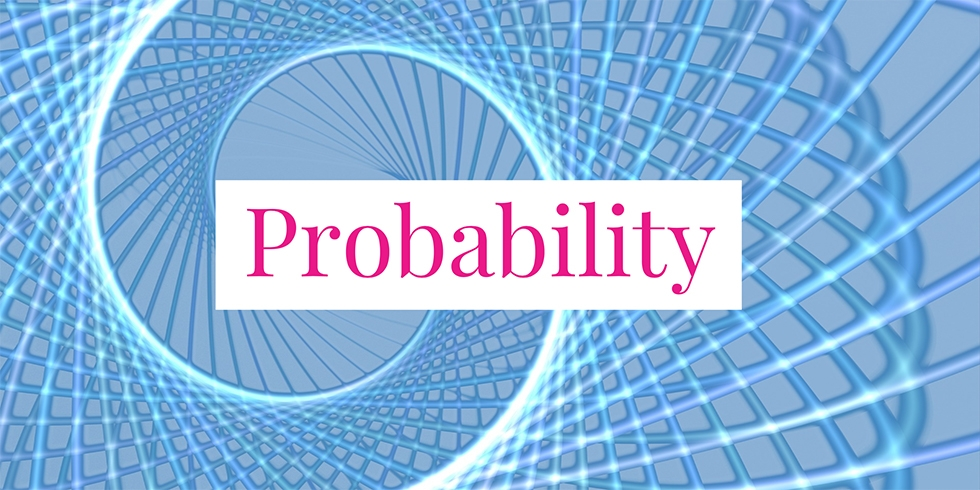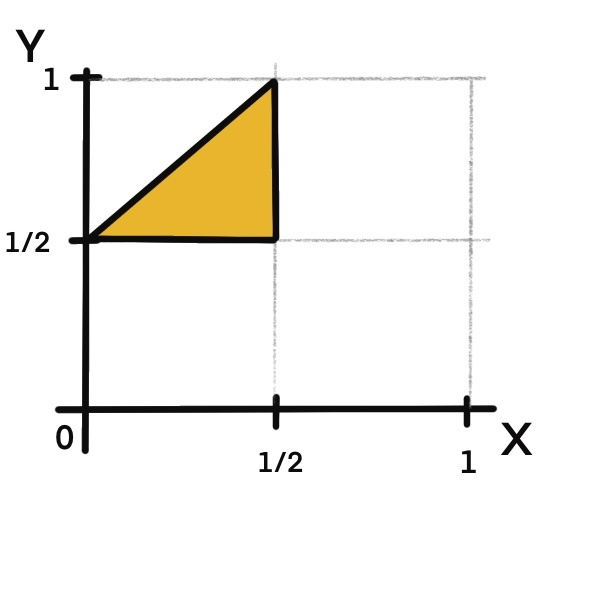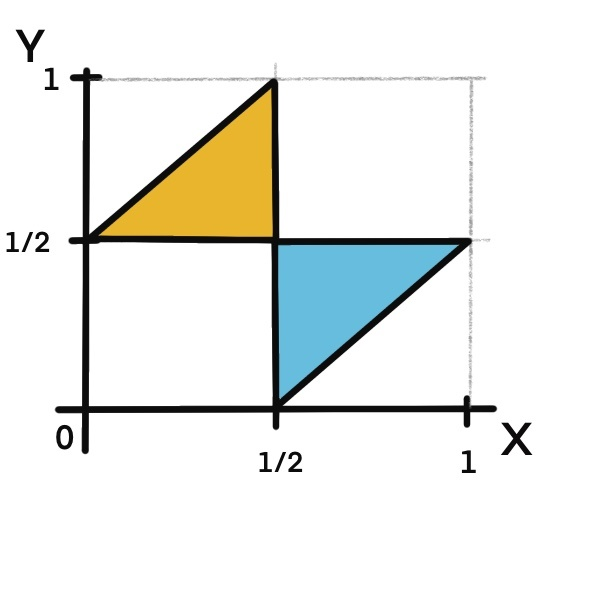# Probability that Three Pieces Form a Triangle## Problem 754

We have a stick of a unit length. Two points on the stick will be selected randomly (uniformly along the length of the stick) and independently. Then we break the stick at these two points so that we get three pieces of the stick. What is the probability that these three pieces form a triangle?Add to solve later

## Solution.

Let us call the left end of the stick the origin. Let $X$ be the length from the origin to the first selected point. Let $Y$ be the length from the origin to the second selected point.

Note that when $X=Y$, we have only two pieces and they cannot form a triangle, so we assume $X \neq Y$.
We first assume that $X < Y$. Then after breaking the stick, we have three pieces of length $X$, $Y-X$, and $1-Y$, respectively.Now, let us consider a condition so that three segments of length $a$, $b$, and $c$ form a triangle in general. It is necessary and sufficient that the sum of the lengths of any two segments is bigger than the length of the rest to form a triangle.
Namely, we must satisfy all of the following inequalities.
\begin{align*}
a + b &> c\\
b + c &> a\\
c + a &> b.
\end{align*}

Applying this condition to the current situation, we must have
\begin{align*}
X + (Y-X) &> 1-Y\\
(Y-X) + (1-Y) & > X\\
(1- Y) + X &> Y – X.
\end{align*}
Simplifying these, we obtain
\begin{align*}
Y &> \frac{1}{2}\\
X &< \frac{1}{2}\\ Y &< X + \frac{1}{2}. \end{align*} The region in the unit square satisfying these inequality is depicted in the figure below (orange region).Note that the joint density function of $X$ and $Y$ are uniformly distributed on the unit square. Thus, the probability that three pieces form a triangle under the assumption $X < Y$ is given by the area of the orange triangular region, which is $1/8$. Note that by symmetry, the case $X > Y$ gives the same probability. Hence, the desired probability is
\begin{align*}
&P(\text{form a triangle}) \\
&= P(\text{form a triangle} \mid X < Y) + P(\text{form a triangle} \mid X > Y)\\
&\frac{1}{8}+\frac{1}{8} = \frac{1}{4}.
\end{align*}

Alternatively, we can find the probability for the case $X > Y$ as follows. By symmetry argument, we simply need to exchange $X$ and $Y$ and obtain the conditions
\begin{align*}
X &> \frac{1}{2}\\
Y &< \frac{1}{2}\\ X &< Y + \frac{1}{2}. \end{align*} The blue region below shows the area surrounded by these inequalities. The area of this blue region is $1/8$. Thus, the total area is $1/8 + 1/8 = 1/4$ as before.Add to solve later

### More from my site

#### You may also like...

This site uses Akismet to reduce spam. Learn how your comment data is processed.

###### More in Probability##### Upper Bound of the Variance When a Random Variable is Bounded

Let $c$ be a fixed positive number. Let $X$ be a random variable that takes values only between $0$ and...

Close